I.1.2

11/26/2020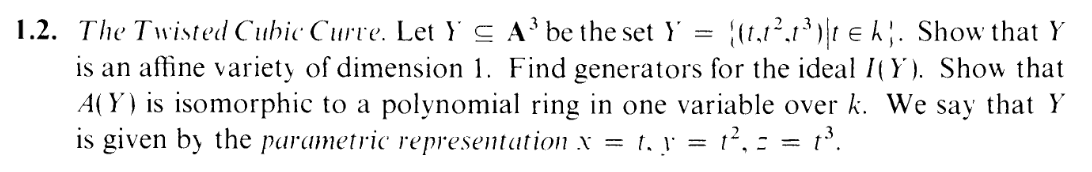Sitting in my room, sitting in my room, screaming into the void in the text. Twisted cubic curve? What? Why? Is there some sort of beauty in this construction that I’m missing? 1-2-3? x-y-z? a-b-c? Yeah right. Mathematicians canappeal to all the tetrahedrons and Koch Curves all they want. I’m not watching your ”I generated Tetris with the Mandelbrot set!” nonsense. Perfect symmetry is ugly. Suck it, nerds. Gimme a construction as exciting as a curvy, dainty human foot and then I might be interested. Hey, Numberphile: how bout you make a video about that one drawing of Suwako lounging by a porch in ”the pose”?

Anyway, this one was actually easy... sort of. A bit of luck helps with this one, but it’s basically the same as 1.1a.

So we need to show 3 things

(1) dimY = 1
(2) Find generators for I(Y )
(3) A(Y ) = k[w]

The way this is ordered is misleading. (1) is actually the thing you want to leave for LAST. Now, let’s start by trying to get (2) (hint, you actually end up getting (3) first, so you prove these in REVERSE order)

Well, looking at the definition of Y, I know the following relations hold:
y = x2
z = x3

Now, I could rewrite that last equation as z=xy. This is actually the more inconvenient choice, as I’ll explain. Let’s just go with z = x3. Then let’s take J = (y - x2,z - x3). By construction, Y Z(J). And it’s easy to show that Z(J) Y . So Y = Z(J).

Taking I of both sides

I(Y ) = I(Z(J)) =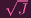.

Now, I would like J to be prime, but from my 1.1a fiasco, I know that I can get that by showing that k[x,y,z]∕J = k[w]. So let’s do that.

so as usual we create a surjective func
f : k[x,y,z] k[w]

whose kernel is J = (y - x2,z - x3)

This was scary at first because there are TWO generators, but we’re just going to double up on the Euclidean division.

let f be a k-alg morph given by
x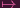w
y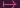w2
z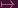w3

So by construction, J kerf.

Now for the reverse inclusion, consider g kerf.

Consider g as an elem of k[x,z][y] and divide by y - x2:

g(x,y,z) = (y - x2)q1(x,y,z) + r1(x,z)

Now, we can’t quite take f() of both sides yet, because if r1 isn’t in terms of just one indeterminate in k[x,y,z], then you can’t do the ”equate the coefficients” trick I did in 1.1a–you’ll leave open the possibility of like terms combining in k[w] to 0 (e.g. g = xz -x4w4 -w4 = 0 even though the coefficients aren't 0). The main point is that we want the remainder to be in terms of just one indeterminate.

Now here’s where choosing z -x3 instead of z -xy comes in handy. You can see r1(x,z) as an elem of k[x][z] and divide it by z - x3, yielding

r1(x,z) = (z - x3)q2(x,z) + r2(x)

So, in total, you have

g(x,y,z) = (y - x2)q1(x,y,z) + (z - x3)q2(x,z) + r2(x)

And NOW, taking f of both sides tells you that r2(x) = 0 and that g (y -x2,z -x3). Same argument as in 1.1a, which has typos but whatever.

(You’re not actually DOOMED if you picked z - xy instead. It’s just that if you’re dividing by that, you have to still consider r1 as an elem of k[x,y][z], so your remainder would come out as r2(x,y) in which case you have to divide by y - x2 again to get rid of the y.)

So J = kerf, so k[x,y,z]∕J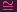k[w], making the left hand side an integral domain, so J is prime, implying that it’s radical and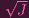= J, meaning that I(Y ) = J, so y - z2,z - x3 ARE generators for I(Y ) (alternatively y - z2,z - xy. so (2) is proven. And we also have that A(Y ) = k[x,y,z]∕J = k[w], so (3) is proven.

Also, since I(Y ) is prime, Y = Z(I(Y )) is an irreducible space, i.e. it’s an affine set. So we can use this proposition from the book: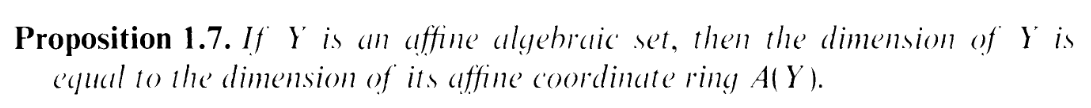So,

dimY = dimA(Y ) = dimk[w] = 1

How do I know that last equality? The book has some justification involving transcendence degrees or something which I forgot the details of. I’m just taking it for granted lmao. So (1) is done.

So yay, that wasn’t too bad. I have no intuition for what just happened and I don’t know why any of this matters, but whatever.

Wait, I feel like I didn’t cuss for this entire post. Let me fix that:

CUNT CUNT CUNT CUNT CUNT CUNT CUNT CUNT CUNT CUNT CUNT CUNT CUNT CUNT CUNT CUNT CUNT CUNT CUNT CUNT CUNT CUNT CUNT CUNT CUNT CUNT CUNT CUNT CUNT CUNT CUNT CUNT CUNT CUNT CUNT CUNT CUNT CUNT CUNT CUNT CUNT CUNT CUNT CUNT CUNT CUNT CUNT CUNT CUNT CUNT CUNT CUNT CUNT CUNT CUNT CUNT CUNT CUNT CUNT CUNT CUNT CUNT CUNT CUNT CUNT CUNT CUNT CUNT CUNT CUNT CUNT CUNT CUNT CUNT CUNT CUNT CUNT CUN T CUNT CUNT CUNT CUNT CUNT CUNT CUN T CNUNT CUNT CUNT CUNT CUNT CUNT CUNT CNTU CUNT CUNT CUNT CUNT CUNT CUNT UCNT CUNT CUNT CUNT CUNT CUNT UCNT CUNT CUNT CUNT CUNT CUNT CUNT CUNT CUNT CUNT CUT CUNT CUNT CUNT CUNT CUNT CUNT CUNT CUNT CUNT CUNT CUNT CUNT CUNT CUNT CUNT CUNT CUNT CUNT CUNT CUNT CUNT CUNT CUNT CUNT CUNT CUNT CUNT CUNT CUNT CUNT

MISC:
At first I used the mathml option with make4ht, and it looked quite nice on Firefox, but then I looked up "mathml" and discovered it's not supported on Chrome, among other things. So this was generated with the default make4ht again.

I also now see I was repeatedly using \in (membership) instead of \subset (subset inclusion) in this post. Woops. You know what I mean.